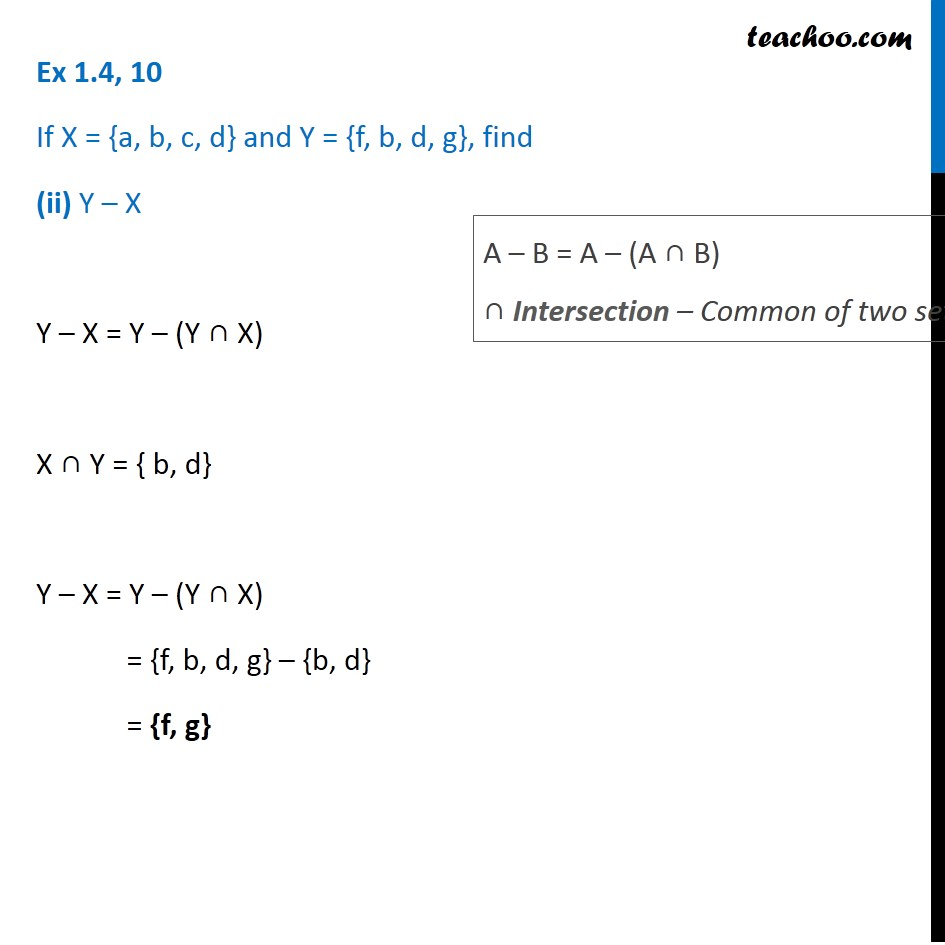Ex 1.4

Chapter 1 Class 11 Sets (Term 1)
Serial order wise### Transcript

If X = {a, b, c, d} and Y = {f, b, d, g}, find (ii) Y – X Y – X = Y – (Y ∩ X) X ∩ Y = { b, d} Y – X = Y – (Y ∩ X) = {f, b, d, g} – {b, d} = {f, g} A – B = A – (A ∩ B) ∩ Intersection – Common of two sets Ex 1.4, 10 If X = {a, b, c, d} and Y = {f, b, d, g}, find